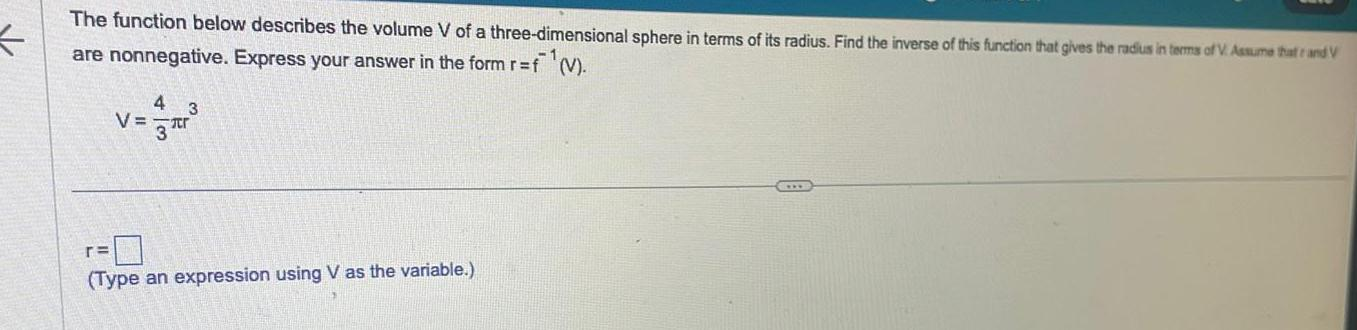Question:

# The function below describes the volume V of a three

Last updated: 11/21/2023The function below describes the volume V of a three dimensional sphere in terms of its radius Find the inverse of this function that gives the radius in terms of V Assume that rand V are nonnegative Express your answer in the form r f V 4 3 v r Type an expression using V as the variable